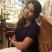# Waves: Overview, Questions, Preparation

Waves 2021

1.2K Views
This chapter has multiple topics listed below:Vipra ShrivastavaSenior Manager - Content

Updated on May 6, 2021 01:42 IST
1. What is the Doppler Effect?
2. Derivation of Doppler Effect
3. Illustrated Examples
4. FAQs on Doppler Effect Derivation

## What is the Doppler Effect?

The Doppler Effect is the relationship between the frequency change in a wave and the observer relative to the wave source. This phenomenon was named after the physicist Christian Doppler in 1842. The most common example of the Doppler Effect is the sound of a car horn receding in the distance when it reaches the observer. The received frequency of the wave is comparatively higher than the emitted frequency.

The Doppler Effect depends upon various factors, including the observer’s motion, the wave source’s motion, hindrance in the air, the motion of the medium, etc. These effects of the waves are studied separately to know the phenomenon of the Doppler Effect.

## Derivation of Doppler Effect

The derivation can be broken down into two main parts,

• When the source is moving.
• When the observer is moving.

To understand both of these effects, let us study in detail.

(i) When the source is moving

Let us understand the effect when the source is moving, and the observer stays in a stationary motion. We assume that the medium is also at rest. Shown below is the illustration of the Doppler Effect when the source is moving:The formula can be derived as:(ii) When the observer is moving

In this case, the observer is in motion, and the source remains at rest, now the derivation happens in the opposite manner and records in the observer’s reference frame. Following the same procedure, we can calculate the first- time travel, as follows:(iii) When both observer and source are moving

There is also a third possibility when both observer and source can be seen moving, the sound wave emits and reaches the observer. The diagrammatic presentation of the same is as follows:Weightage in Class 11

The chapter ‘Waves’ for class 11 holds a weightage of 5 marks. It consists of 2 short questions of 2 marks and 3 marks each.

## Illustrated Examples

1. Provide a diagram example of the Doppler effect when both source and the observer are moving.2. Provide a diagram example showing the Doppler effect when the source is moving.3. State one use of Doppler effect in the medical industry.

Answer: Doppler effect is used to diagnose heart problems.

Image courtesy: NCERT

## FAQs on Doppler Effect Derivation

Q: What’s the origin behind the name Doppler Effect?

A: This phenomenon was named after the physicist Christian Doppler in 1842.

Q: Explain a basic example of the Doppler Effect.

A: The most common example of the Doppler Effect is the sound of a car horn recedes in the distance when it reaches the observer. The received frequency of the wave is comparatively higher than the emitted frequency.

Q: What factors does the Doppler Effect rely upon?

A: The Doppler Effect depends upon various factors, such as the observer’s motion, the motion of the wave source, hindrance in the air, the motion of the medium, etc.

Q: Explain the two derivative parts of the Doppler Effect?

A:

1) When the source is moving.

2) When the observer is moving.

Q: Name one routine activity during which we can observe the Doppler Effect?

A: Traffic police use radar guns as an IOT application that used the Doppler Effect to measure the vehicles’ speed.